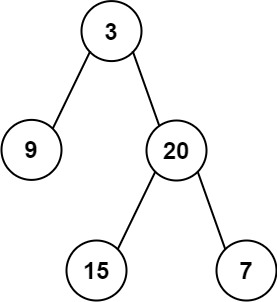## C# || How To Construct Binary Tree From Inorder And Postorder Traversal Using C#The following is a module with functions which demonstrates how to construct a binary tree from in order and post order traversal using C#.

1. Build Tree – Problem Statement

Given two integer arrays inorder and postorder where inorder is the inorder traversal of a binary tree and postorder is the postorder traversal of the same tree, construct and return the binary tree.

Example 1:``` Input: inorder = [9,3,15,20,7], postorder = [9,15,7,20,3] Output: [3,9,20,null,null,15,7] ```

Example 2:

``` Input: inorder = [-1], postorder = [-1] Output: [-1] ```

2. Build Tree – Solution

The following is a solution which demonstrates how to construct a binary tree from in order and post order traversal.

``` 2. Build Tree - Solution C# // ============================================================================ // Author: Kenneth Perkins // Date: Nov 20, 2021 // Taken From: http://programmingnotes.org/ // File: Solution.cs // Description: Demonstrates how to build binary tree from in order and post order // ============================================================================ /** * Definition for a binary tree node. * public class TreeNode { * public int val; * public TreeNode left; * public TreeNode right; * public TreeNode(int val=0, TreeNode left=null, TreeNode right=null) { * this.val = val; * this.left = left; * this.right = right; * } * } */ public class Solution { private int postorderIndex; private Dictionary<int, int> inorderIndexMap; public TreeNode BuildTree(int[] inorder, int[] postorder) { postorderIndex = postorder.Length - 1; // Build a map to store value & its index relation inorderIndexMap = new Dictionary<int, int>(); for (int index = 0; index < inorder.Length; ++index) { inorderIndexMap[inorder[index]] = index; } return ArrayToTree(postorder, 0, postorder.Length - 1); } private TreeNode ArrayToTree(int[] postorder, int start, int end) { // If there are no elements to construct the tree if (start > end) { return null; } // Select the postorder element as the root and decrement it var rootValue = postorder[postorderIndex--]; var root = new TreeNode(rootValue); // Get current positon var curPos = inorderIndexMap[rootValue]; // Build left and right subtree // excluding inorderIndexMap[rootValue] element because it's the root root.right = ArrayToTree(postorder, curPos + 1, end); root.left = ArrayToTree(postorder, start, curPos - 1); return root; } }// http://programmingnotes.org/ 12345678910111213141516171819202122232425262728293031323334353637383940414243444546474849505152535455 // ============================================================================//    Author: Kenneth Perkins//    Date:   Nov 20, 2021//    Taken From: http://programmingnotes.org///    File:  Solution.cs//    Description: Demonstrates how to build binary tree from in order and post order// ============================================================================/** * Definition for a binary tree node. * public class TreeNode { *     public int val; *     public TreeNode left; *     public TreeNode right; *     public TreeNode(int val=0, TreeNode left=null, TreeNode right=null) { *         this.val = val; *         this.left = left; *         this.right = right; *     } * } */public class Solution {    private int postorderIndex;    private Dictionary<int, int> inorderIndexMap;     public TreeNode BuildTree(int[] inorder, int[] postorder) {        postorderIndex = postorder.Length - 1;         // Build a map to store value & its index relation        inorderIndexMap = new Dictionary<int, int>();        for (int index = 0; index < inorder.Length; ++index) {            inorderIndexMap[inorder[index]] = index;        }        return ArrayToTree(postorder, 0, postorder.Length - 1);    }     private TreeNode ArrayToTree(int[] postorder, int start, int end) {        // If there are no elements to construct the tree        if (start > end) {            return null;        }         // Select the postorder element as the root and decrement it        var rootValue = postorder[postorderIndex--];        var root = new TreeNode(rootValue);         // Get current positon        var curPos = inorderIndexMap[rootValue];         // Build left and right subtree        // excluding inorderIndexMap[rootValue] element because it's the root        root.right = ArrayToTree(postorder, curPos + 1, end);        root.left = ArrayToTree(postorder, start, curPos - 1);        return root;    }}// http://programmingnotes.org/ ```

QUICK NOTES:
The highlighted lines are sections of interest to look out for.

The code is heavily commented, so no further insight is necessary. If you have any questions, feel free to leave a comment below.

Once compiled, you should get this as your output for the example cases:

``` [3,9,20,null,null,15,7] [-1] ```